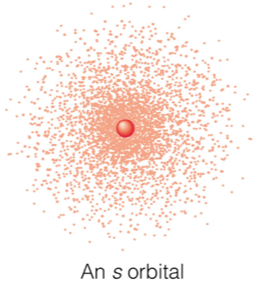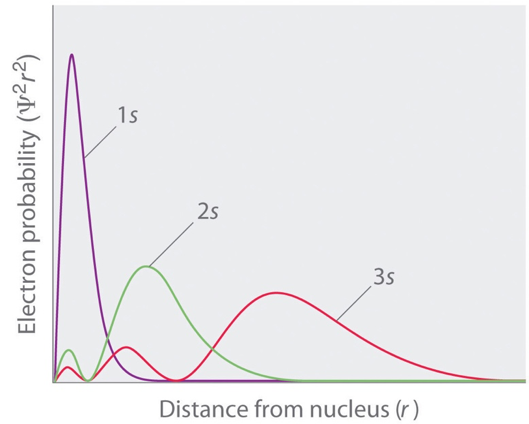# Schrödinger's Contribution to The Atomic Model

This topic is part of the HSC Physics course under the section Quantum Mechanical Nature of the Atom.

### HSC Physics Syllabus

• analyse the contribution of Schrödinger to the current model of the atom

### Schrödinger’s Contribution to the Current Model of the Atom

Schrödinger used de Broglie’s matter wave theory to develop a probabilistic model of the atom.

In Schrödinger's model, electrons do not follow sharply defined orbits (like in Bohr's model), but rather are found in orbitals. In addition, Schrödinger's atomic model is based on the quantum mechanical and wave nature of electrons, both of which are described in equations called wave functions

Orbitals are regions in which an electrons can be found. Inside an orbital, the probability of finding an electron is described by mathematical equations proposed by Schrödinger.

Unlike orbits, orbitals are visual models of the probability density of electrons in an atom. While 'orbits' and 'orbitals' seem to share similarities, they are very different concepts.

Different types of orbitals have different shapes and therefore electron density patterns.Illustration of the 1s orbital depicts electron density surrounding a red nucleus in the centre. Appreciate the evident increase in probability of finding an electron with proximity to the nucleus.

### Quantum Numbers

Quantum numbers describe the quantum state of an electron. There are four quantum numbers, each providing information on a different aspect of an electron's quantum state.

Principal quantum number (n) determines the size and energy of orbital an electron resides in. The energy of an orbital increases the further it is from the nucleus.Illustrations depict different perspectives of s orbitals. Figures in the first row show a three-dimensional model of s orbitals. Appreciate the presence of nodes between s orbitals. Figures in the second row show the same features in a two-dimensional manner.

For example, the energy and size of s orbitals increase as the principal quantum number increases. 2s orbital has greater energy and is further from the nucleus than 1s orbital.

Orbitals of various energies are separated by ﻿nodes﻿ where electrons are forbidden. This feature of the model is consistent with the quantum mechanical nature of electrons as they must belong to orbitals of specific energies.Wave function squared versus distance from the nucleus for s orbitals.

The square of an electron's wave function provides an equation describing its probability density for a given orbital. When the square of the wave function is plotted against the distance from the nucleus, it gives rise to the graph above.

Angular quantum number determines the shape of the orbital an electron is found in. Orbitals of different shapes are denoted by different letters: s, p, d and fMagnetic quantum number determines the orientation of each orbital in three dimensional space. For example, the p orbital can be orientated in three ways (shown above).

Spin quantum number determines whether an electron has a up or down spin. Electrons in each specific orbital must have opposing spins.

### Pauli's Exclusion Principle

Pauli's exclusion principle states that no two electrons in an atom can share the same set of four quantum numbers. In other words, an electron must have its own unique combination of quantum numbers.

Previous section: de Broglie's Matter Waves